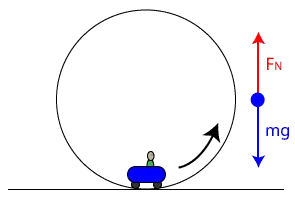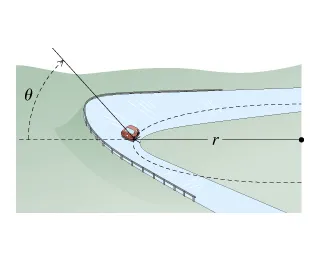# Physics - Classical mechanics - Circular dynamic and vertical circle motion examplesHello it's a me! Today we continue with some more examples in Physics.

The last two posts where about examples in:

Object equilibrium and 2nd law applications

Contact forces and friction

Today's topic will be circular dynamics and vertical circle motion examples.

The theory for that can be found here and I highly suggest you to check it out!

So, without further do, let's get started!

### Example 1 (flat curve):Suppose we have a flat/horizontal curve on a highway with an radius (r) of 200m. A car takes this corner with a velocity of 20 m/s. Which is the least possible (static) friction factor μs so that the car doesn't slide out?

In constant velocity circular motions we know that the acceleration is a = u^2/r.

That way from the second law we have:

ΣF = F = ma = mu^2/r =>

F = m*20^2/200 =>

F = 2m N

The maximum static friction is also equal to:

F = μs*Ν = μmg = 10μm N

By setting those equations equal to each other we get:

2m = 10μsm =>

μs = 0.2

### Example 2 (banked curve):Suppose that we now have a banked curve on a highway with an radius (r) of 350m that will be constructed with an angle (θ). A car needs to be able to take this corner with a velocity of 25 m/s and the friction is theoretically supposed as zero (non-existent). Which is the least angle (θ) so that this can happen?

In my theory post we already found the equations but here a small explanation

The vertical ground force has an angle θ to the gravity and so we find the components:

Nsinθ and Ncosθ (one for each axis)

Using the 1st and 2nd law for each axis respectively we get:

N * sinθ = mu^2 / r    (2nd law)

and

N * cosθ = mg   (1st law)

By dividing these equations we get:

tanθ = u^2/gr =>

θ = arctan(u^2/gr) => θ = arctan(25^2/3500) ~= arctan(0.1785) =>

θ ~= 10.12 degrees

### Example 3(vertical circle):

The radius (r) of a theme park wheel is 9m and it does a circle in 12 seconds.

Calculate the phenomenal weight of an 80kg passenger at the highest and lowest point of the wheel.

Because the period is T = 12 seconds we can calculate the velocity from:

u = 2πr / T  => u ~= 4.71 m/s

The centripetal force Fc stays the same and so:

Fc = mu^2/r => Fc != 197 N

Because the gravity also stays the same and is W = mg = 800 N we know that there is a upward force at the top that is declining the gravity so that:

Fc = W - Ft = mu^2 / r => Ft = W - Fc =>

Ft = 800 - 197 = 603 N (upwards)

This force is acting like a vertical ground force and so the phenomenal weight the top is 603N!

In the same way at the bottom there is a upward force that is also declining gravity so that:

Fc = Fb - W = mu^2 / r => Fb = W + Fc =>

Fb = 197 + 800 = 997N (upwards)

So, the phenomenal weight at the bottom is 997N!

Another interesting topic is how we generate artificial gravity by rotating a space station in space!

### Image sources:And this is actually it and I hope that you learned something!
Next time we will get into more advanced Newton law examples and after that we are finished with everything that I wanted to cover about Classical mechanics (for now)! This means that we will get into a new physics branch!

Bye!

H2
H3
H4
3 columns
2 columns
1 column
Join the conversation now